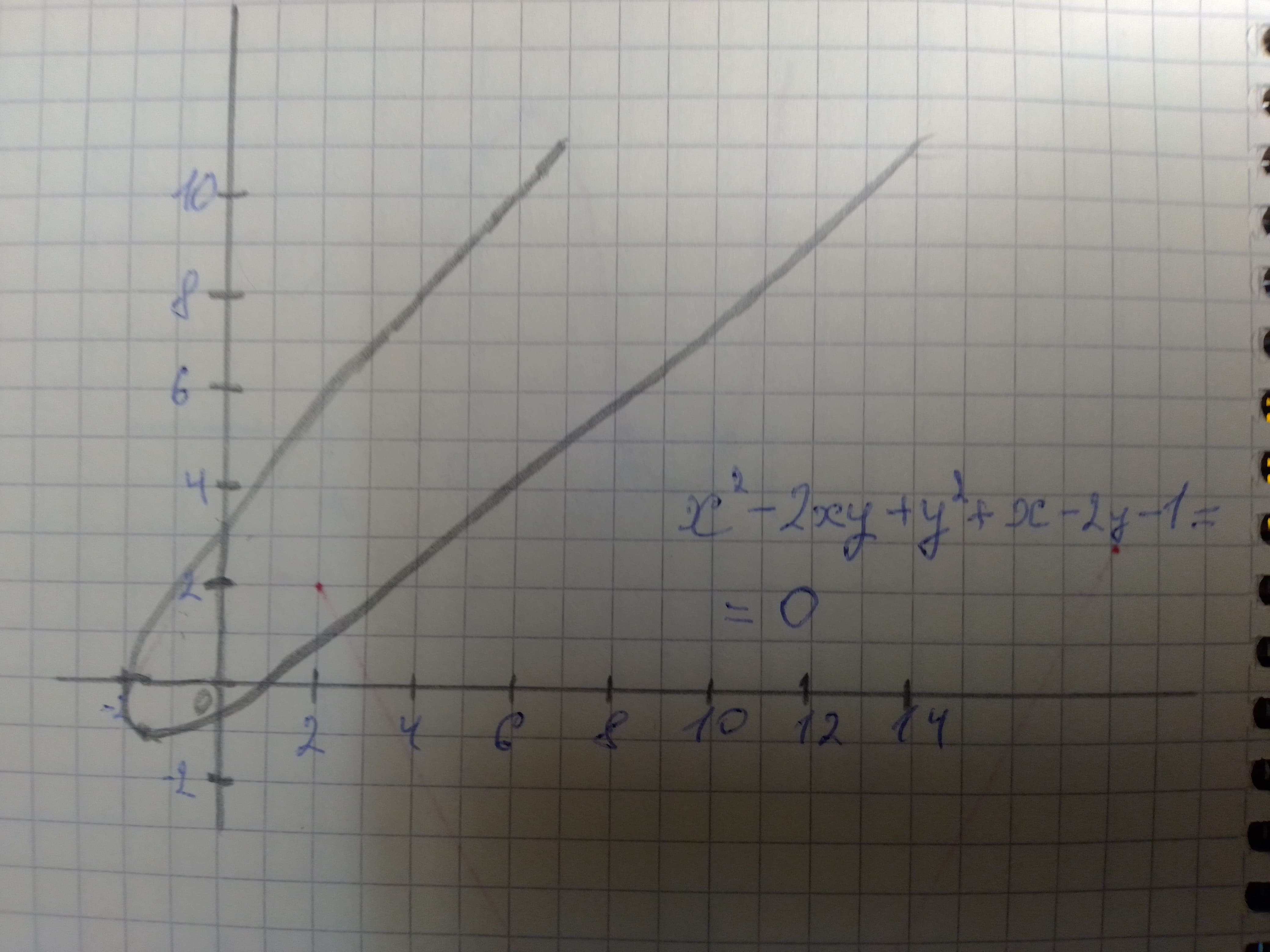# Given the following conic section, determine its shape and then sketch its graph. displaystyle{x}^{2}-{2}{x}{y}+{y}^{2}+{x}-{2}{y}-{1}={0}Suman Cole 2021-02-22 Answered
Given the following conic section, determine its shape and then sketch its graph.
${x}^{2}-2xy+{y}^{2}+x-2y-1=0$
You can still ask an expert for help

• Questions are typically answered in as fast as 30 minutes

Solve your problem for the price of one coffee

• Math expert for every subject
• Pay only if we can solve itlamusesamuset

Step 1
We need to determine the shape of the given conic section using its equation.
${x}^{2}-2xy+{y}^{2}+x-2y-1=0$
Step 2
We know that, for the general equation of conic section
$A{x}^{2}+Bxy+C{y}^{2}+Dx+Ey+F=0$
if ${B}^{2}-4AC=0,$ then the conic must be a parabola.
For the given equation, $A=1,B=-2,C=1$
${B}^{2}-4AC={\left(-2\right)}^{2}-4\left(1\right)\left(1\right)$
$=0=>$ given conic section is a parabola
Step 3
The graph of the parabola has been shown below.